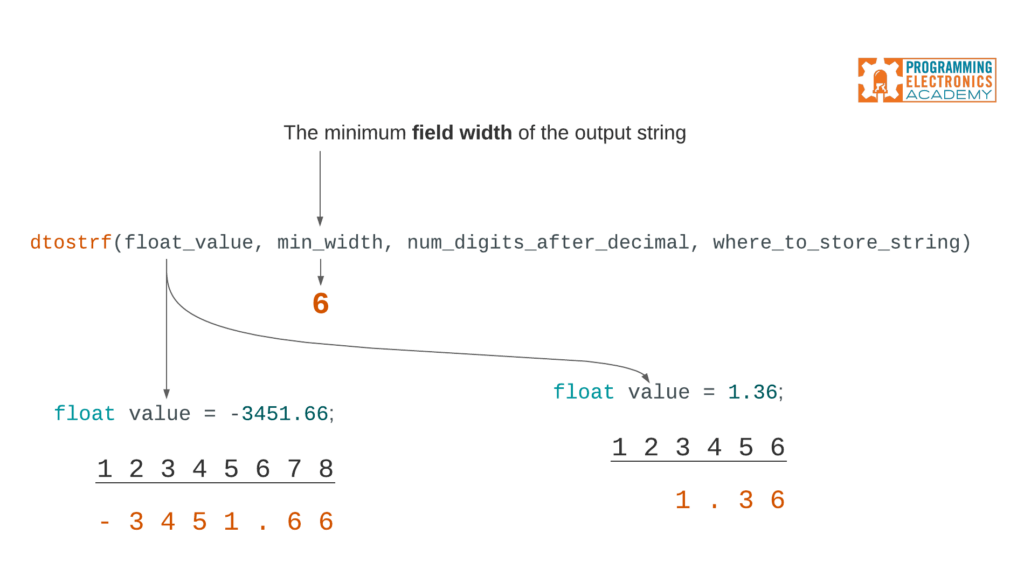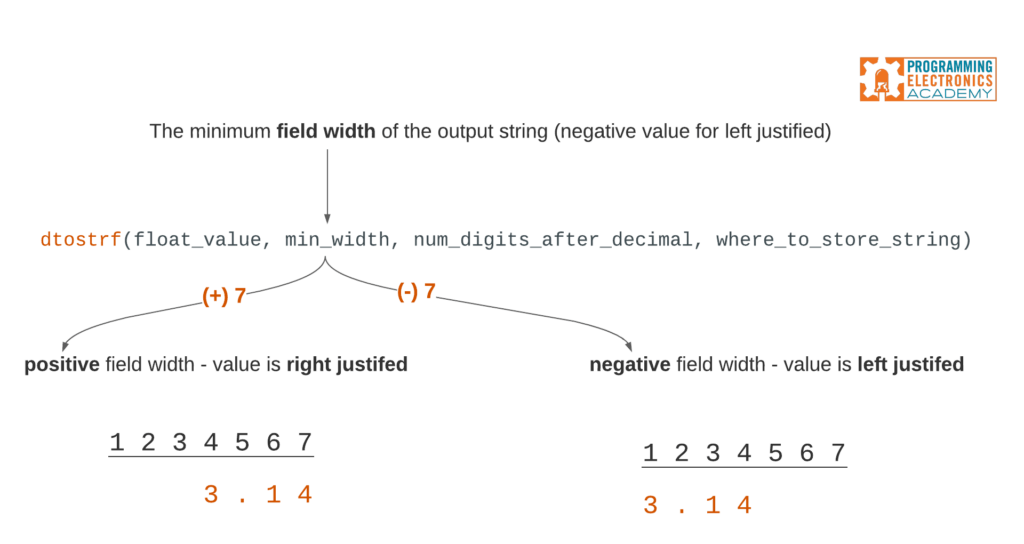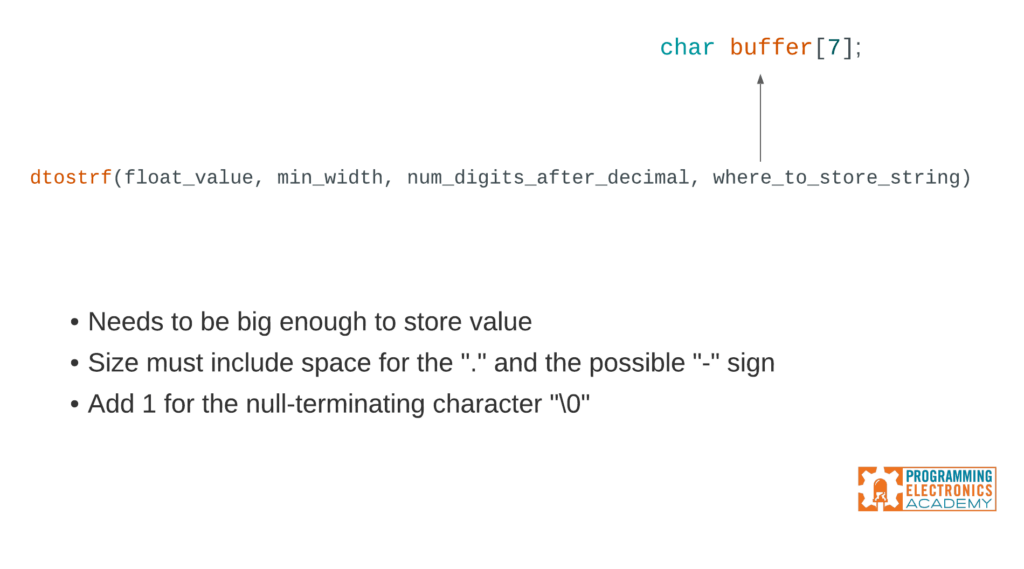# dtostrf() turn your floats into strings

### Содержание

dtostrf() may be the function you need if you have a floating point value that you need to convert to a string.

In this lesson you will learn exactly how to use dtostrf in your Arduino code.## dtostrf() syntax

Let’s jump straight into this. Here are the parameters that dtostrf() expects:The first value is the floating point number that you want to convert into a string that’s easy enough.## minimum field width

The second value is the minimum field width.

If you set the minimum field width to 6, and you convert a floating point value that has greater than 6 digits, those extra digits will still display. And for counting purposes, the “.” decimal point and the “-” negative sign count as spaces too.

If you set the minimum field width to 6, and you convert a floating point value that has less than 6 digits, then spaces will be added before the number starts.The minimum field width can also be negative. If you pass dtostrf() a negative value, it will left-justify the number within the field width.## precision

The 3rd argument passed to dtostrf() is the precision, which is the number of digits to show after the decimal point. If the floating point value you convert to a string has more digits after the decimal point than the number specified in the precision, then it will be cut off and rounded accordingly in the output string.

If you pass a number with fewer digits after the decimal point, it will add trailing zeros to the number.## dtostrf() char buffer sizing

The final argument passed to dtostrf() is where you want to store the output string. This will typically be a character array, like buffer. When determining the size of this buffer, make sure to consider:

• What the biggest number might ever be
• Size must include space for the &#8220;.&#8221; and the possible &#8220;-&#8221; sign
• Add 1 for the null-terminating character &#8220;\0&#8221;## Let Us know

What are you using dtostrf() for? We&#8217;d love to know tell us in the comments! Also, if you found this useful, you might also find our sprintf() lesson super handy as well.

Источник: www.programmingelectronics.com